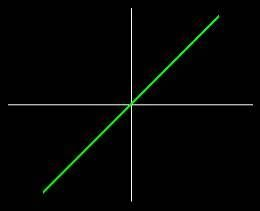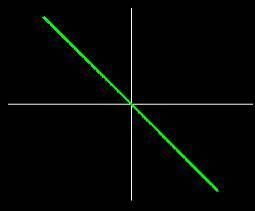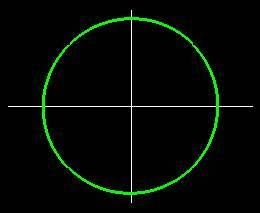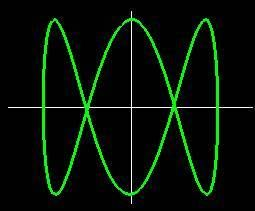# Lissajous Figures

## Introduction

When using an oscilloscope, we can plot one sinusoidal signal along the x-axis against another sinusoidal signal along the y-axis. The result is a Lissajous figure. Lissajous figures tells us about the phase difference between the two signals and the ratio of their frequencies. Five such figures are shown in figures 1-5 below.Fig. 1: Two sine waves of equal frequency, in phase.Fig. 2: Two sine waves of equal frequency, 180 degrees out of phase.Fig. 3: Two sine waves of equal frequency, 90 degrees out of phase.Fig. 4: Two sine waves, in phase, frequency of horizontal wave twice frequency of vertical wave.Fig. 5: Two sine waves, in phase, frequency of horizontal wave three times frequency of vertical wave.